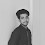# URI / BEE CROWD 1071 - Sum of Consecutive Odd Numbers I Solution in C,C++,Python

## URI / BEE CROWD 1071 - Sum of Consecutive Odd Numbers I Solution in C,C++,Python | URI Online Judge Solution 1071 - Sum of Consecutive Odd Numbers I

Read two integer values and Y. Print the sum of all odd values between them.

## Input

The input file contain two integer values.

## Output

The program must print an integer number. This number is the sum off all odd values between both input values that must fit in an integer number.

 Sample Input Sample Output 6-5 5
 1512 13
 1212 0

### Demonstration:

Any integer that cannot be divided exactly by 2 is an odd number.
Here we apply a range-based while loop which will run between two numbers and checking the condition for Sum of Consecutive Odd Numbers I are applied and printing the total sum of odd numbers.
N.B: Don't copy paste the code as same. Just try to understand it and try yourself.

### URI Problem 1071 Solution in C :

 URI Online Judge 1071 Solve  in C :
```#include <stdio.h>

int main()
{
int x, y, sum = 0, i;
int min, max;

scanf("%d%d", &x,&y);

if(x < y){
min = x;
max = y;
}else{
max = x;
min = y;
}

for(i = (min + 1); i < max; ++i)
{
if(i % 2 != 0){
sum += i;
}
}

printf("%d\n", sum);

return 0;
}
```

### 5 Responses to URI / BEE CROWD 1071 - Sum of Consecutive Odd Numbers I Solution in C,C++,Python

1.2.Thanks,Stay with us❤️

3.Hi brother, Will you continue solving URI problems ? U haven't posted in this section for a long time.

4.I will continue solving URI / BEE problems from next months. Thanks for supporting me.

5.#include
int main(){
int a,b,i;
int sum=0;
scanf("%d %d",&a,&b);
if(a>b){
for(i=(b+1); i<a; i++){
if(i%2!=0){
sum=sum+i;
}

}
}else{
for(i=(a+1); i<b; i++){
if(i%2!=0){
sum=sum+i;
}
}
}
printf("%d\n",sum);
return 0;
}